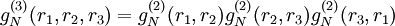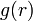# Difference between revisions of "Kirkwood superposition approximation"

Jump to: navigation, search

John G. Kirkwood 1935 (Eq. 40 Ref. 1, Eq. 5.6 Ref. 2)$g_N^{(3)}(r_1,r_2,r_3)=g_N^{(2)}(r_1,r_2)g_N^{(2)}(r_2,r_3)g_N^{(2)}(r_3,r_1)$

It appears that this was used as a basis of a closure for the Kirkwood integral equation (Ref. 1) and the Yvon, and Born-Green (Ref. 2) until the work of Morita and Hiroike (Ref. 3). It was pointed out in Ref.s 4 and 5, that there is an inconsistency between the pressure and the compressibility equation if this superposition approximation is used to generate$g(r)$.